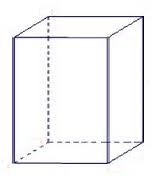Calculate the volume (V) and the surface (S) of a regular quadrilateral prism whose height is 28.6 cm and the deviation of the body diagonal from the base plane is 50°.

V =  8235.5985 cm3
S =  2517.2067 cm2

### Step-by-step explanation:Did you find an error or inaccuracy? Feel free to write us. Thank you!Tips to related online calculators
Tip: Our volume units converter will help you with the conversion of volume units.

#### You need to know the following knowledge to solve this word math problem:

We encourage you to watch this tutorial video on this math problem:

## Related math problems and questions:The height of a regular quadrilateral prism is v = 10 cm, the deviation of the body diagonal from the base is 60°. Determine the length of the base edges, the surface, and the volume of the prism.
• Four sided prismCalculate the volume and surface area of a regular quadrangular prism whose height is 28.6cm and the body diagonal forms a 50-degree angle with the base plane.
• Angle of diagonalThe angle between the body diagonal of a regular quadrilateral and its base is 60°. The edge of the base has a length of 10cm. Calculate the body volume.In a regular quadrilateral pyramid, the height is 6.5 cm and the angle between the base and the side wall is 42°. Calculate the surface area and volume of the body. Round calculations to 1 decimal place.The regular quadrilateral pyramid has a base edge a = 1.56 dm and a height h = 2.05 dm. Calculate: a) the deviation angle of the sidewall plane from the base plane b) deviation angle of the side edge from the plane of the base
• Prism diagonalThe body diagonal of a regular square prism has an angle of 60 degrees with the base, the edge length is 10 cm. What is the volume of the prism?
• Tetrahedral pyramidCalculate the regular tetrahedral pyramid's volume and surface if the content area of the base is 20 cm2, and the deviation angle of the side edges from the plane of the base is 60 degrees.
• Space diagonal anglesCalculate the angle between the body diagonal and the side edge c of the block with dimensions: a = 28cm, b = 45cm and c = 73cm. Then, find the angle between the body diagonal and the plane of the base ABCD.
• Regular square prismThe volume of a regular square prism is 192 cm3. The size of its base edge and the body height is 1: 3. Calculate the surface of the prism.
• Base diagonalIn a regular 4-sided pyramid, the side edge forms an angle of 55° with the base's diagonal. The length of the side edge is eight meters. Calculate the surface area and volume of the pyramid.
• Pyramid 8Calculate the volume and the surface area of a regular quadrangular pyramid with the base side 9 cm and side wall with the base has an angle 75°.
• Cuboid diagonalCalculate the volume and surface area of the cuboid ABCDEFGH, which sides a, b, c has dimensions in the ratio of 9:3:8. If you know that the diagonal wall AC is 86 cm, and the angle between AC and space diagonal AG is 25 degrees.Calculate the volume and surface area of a regular quadrangular prism 35 cm high and the base diagonal of 22 cm.Calculate the volume and surface of 4 sides regular pyramid whose base edge is 4 cm long. The angle from the plane of the sidewall and base plane is 60 degrees.The base of the square prism is a rectangle with dimensions of 3 dm and 4 dm. The height of the prism is 1 m. Find out the angle between the body diagonal with the diagonal of the base.What is the volume of a quadrilateral oblique prism with base edges of length a = 1m, b = 1.1m, c = 1.2m, d = 0.7m, if a side edge of length h = 3.9m has a deviation from the base of 20° 35' and the edges a, b form an angle of 50.5°.How many glass are needed to produce glass with base regular 5-gon if one base triangle in the base is 4.2 square cm and the height is 10 cm?